Compare Brokers

Vs.

# What is a Pip in Forex Trading?

By Stefano Treviso , Updated on: Apr 07 2023.

A Pip is the smallest price measurement change in forex trading. In most currency pairs one Pip equals a movement in the fourth decimal place (0.0001) with the exception of the JPY where a pip equals a movement in the second decimal place (0.01).Pip is an abbreviation for “percentage in point”.

The reason for traders calling it “percentage in point” is that a Pip is equivalent to 1/100 of 1% (1% of 1%).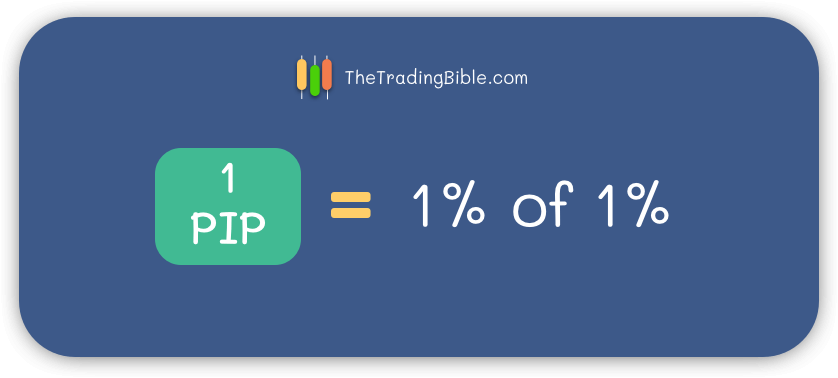To clarify this concept a little bit more, all we need to do is understand that 1% can also be written as 0.01, in fact, if you wanted to know how much is 1% of \$2.000 you can do so by multiplying \$2.000 times 0.01, the result will be \$20.

Now that we understand the concept of 1%, we can follow with obtaining the result of 1/100 of 1% (same as 1% of 1%). To do so, we can simply multiply 0.01 times 0.01, the result is 0.0001 and that is exactly what 1 “percentage point” represents (also known as Basis point).

As mentioned above we know that a Pip can be either 0.0001 for most currencies or 0.01 in the case of JPY pairs, yet there are some brokers that will instead show 5 decimal positions for most currencies and 3 for JPY pairs and here comes into play the “pipette”.

## What is a Pipette (Fractional Pip)?

A pipette is simply 1/10 of a Pip which equals the 5th decimal position for most currency pairs or the 3rd decimal position for pairs that involve the JPY.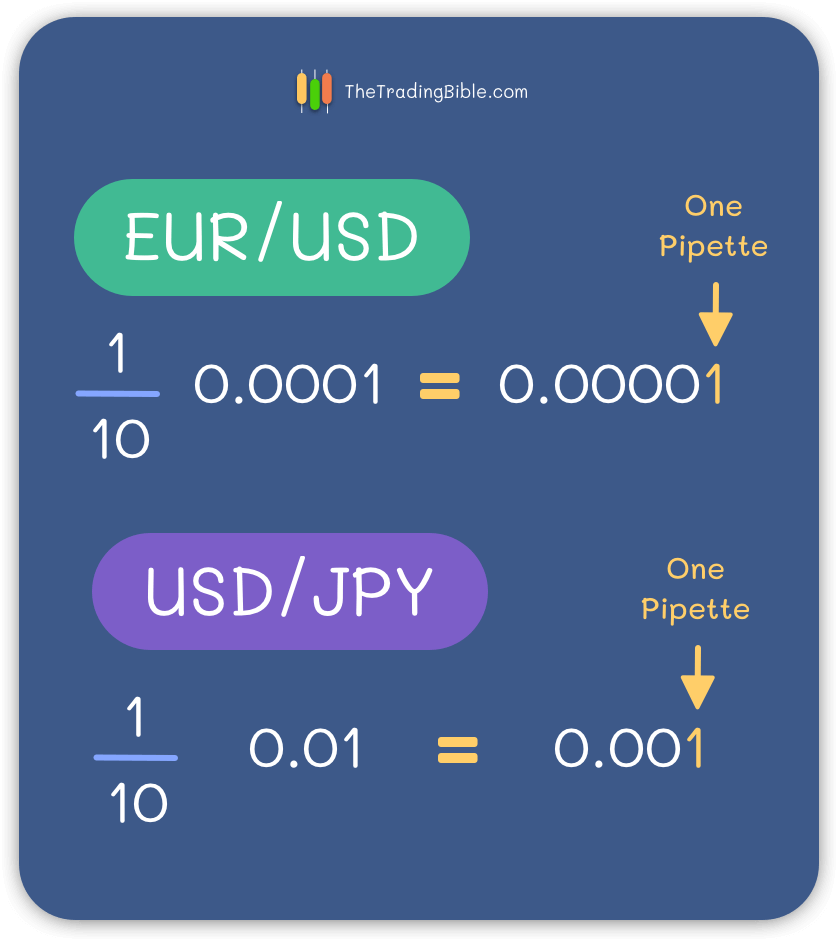To arrive at the correct result simply divide 1 by 10 and multiply it by 1 Pip (0.0001), the result is 0.00001.

## Featured Low Spreads Forex Brokers74% of retail CFD accounts lose money.

Dynamic - From 1 Pip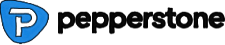74-89% of retail CFD accounts lose money.

Dynamic - From 0 to 0.77 Pips

## Examples of using Pips in Forex

### Case 1: EUR/USD - 4 Decimals

In most currency pairs such as EUR/USD or USD/GBP, a pip equals a movement of 0.0001 (fourth decimal position).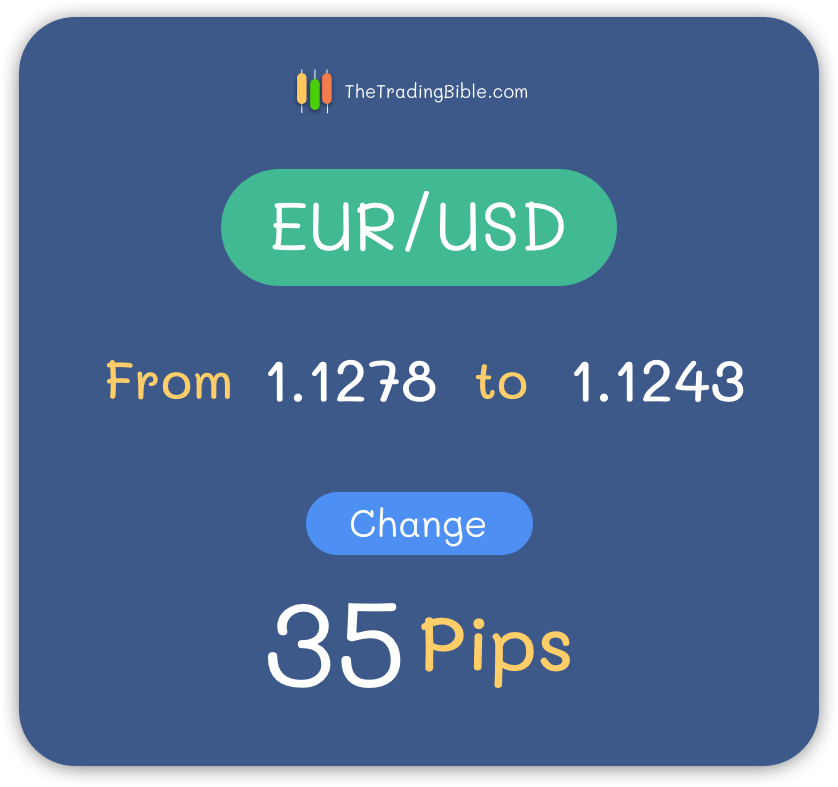To find out how many pips your currency pair changed, simply subtract one price from the other (1.1278 - 1.1243) and in this particular case the result is 35 Pips.

### Case 2: EUR/USD - 5 Decimals

In the case of Brokers that quote most of their currency pairs with 5 decimals, we learned that 0.00001 is called a “Pipette” or 1/10th of a Pip (or 0.1 Pip as well).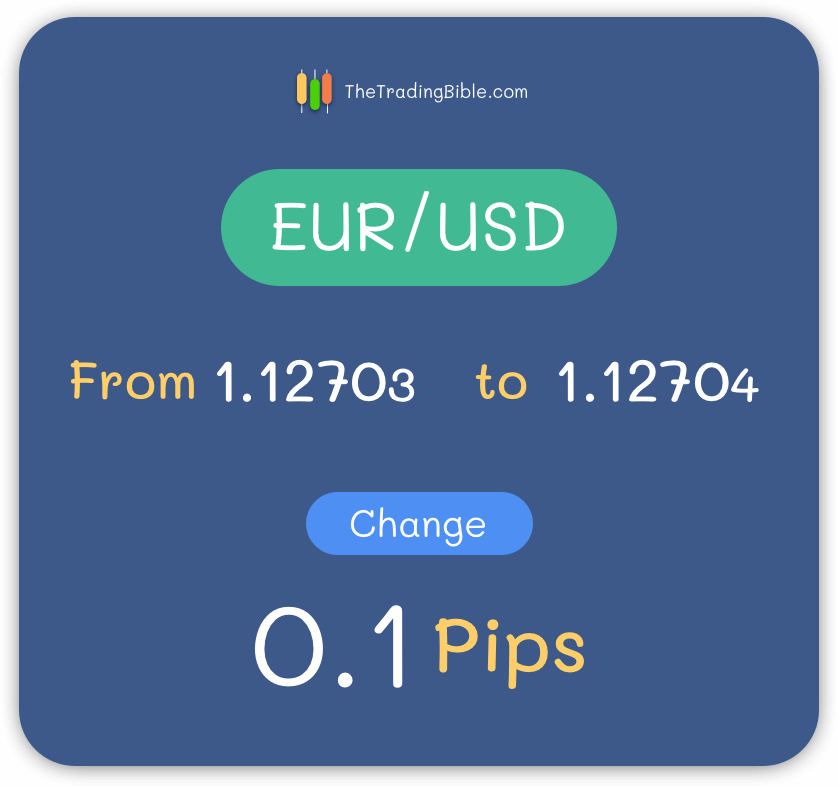To find out the result of how many pips your currency pair changed, subtract both numbers (1.12703 - 1.12704), the result will be 0.00001 (which equals 0.1 Pips).

### Case 3: USD/JPY - 2 Decimals

In most pairs involving the JPY, a pip equals a movement of 0.01 (second decimal position).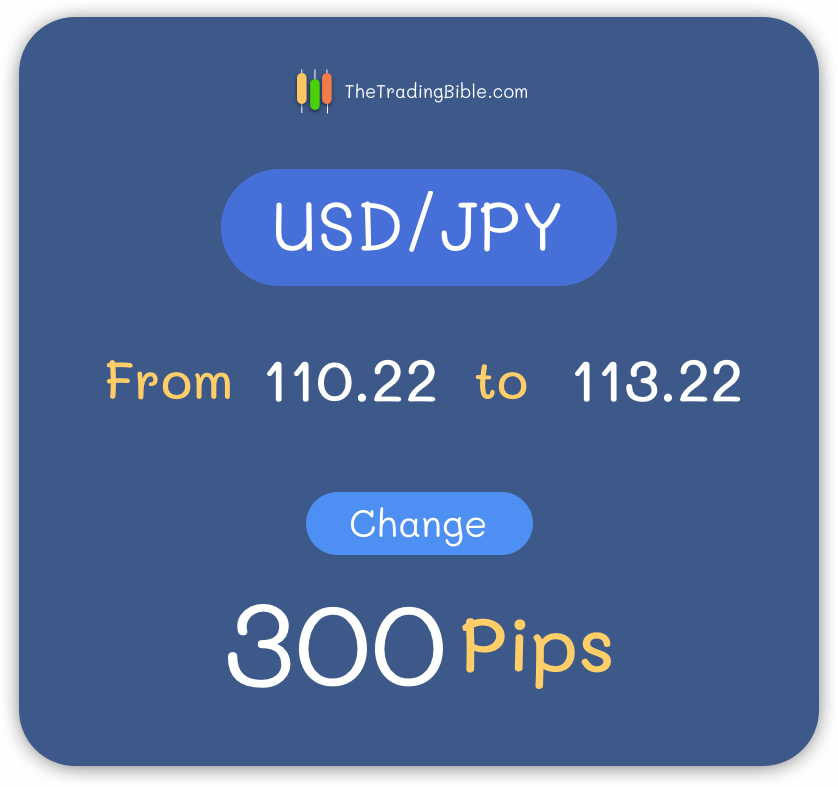To find out the result of how many pips your currency pair changed, subtract both prices (110.22 - 113.22), the result will be 3. In order to arrive at the conclusion that our result equals 300 pips, we need to ask ourselves the following: if 1 Pip equals 0.01 then how many pips is required to arrive at the result of 3?

1. To verify that in the beginning of your calculation simply take your result “3” and divide it by one Pip’s value (0.01), the result will be 300.

### Case 4: USD/JPY - 3 Decimals

In the case of brokers that chose to quote JPY pairs with 3 decimals, we learned that the third decimal position is called a “Pipette” which equals to 1/10th of a Pip (or 0.1 Pips as well).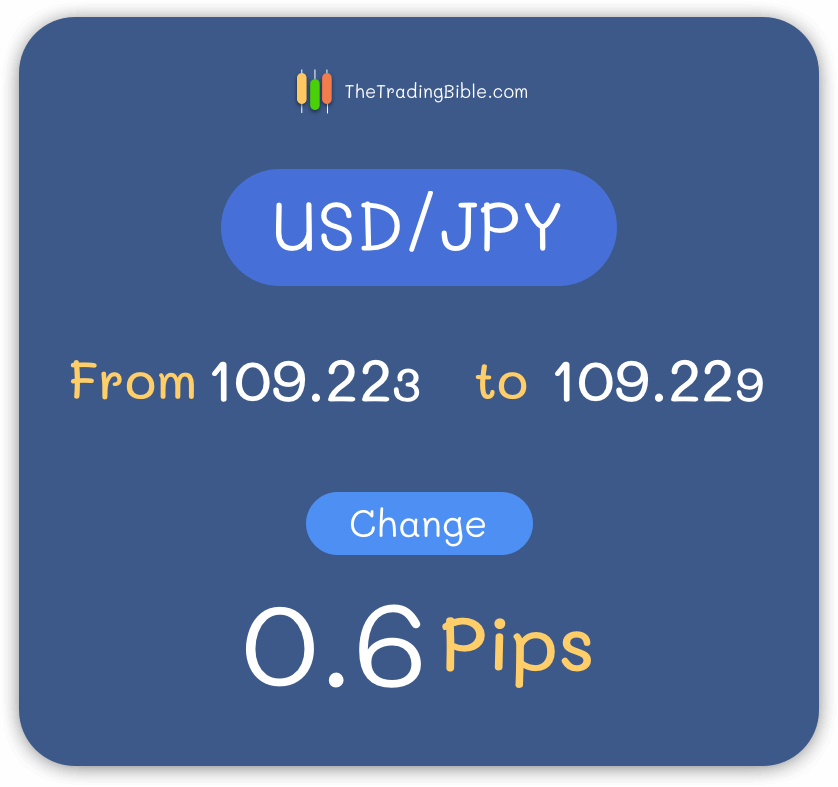To find out the result of how many pips your currency pair changed, subtract both prices (109.223 - 109.229), the result is 0.6 Pips.

## Calculating Pip Value

Now that we understand the basics of how to work with Pips and Pipettes, we can get into calculating their value, which means to understand how much is each Pip worth (in terms of money) in relation to the size of your trade.

A good example of this is asking yourself the question: do every time my open trade changes 1 Pip, how much am I profiting or losing \$1, \$10, \$100?

Let's get started with the formula to arrive at this result: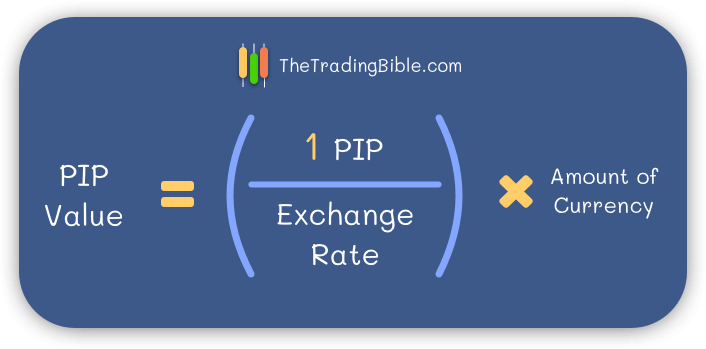The above formula is very simple, let’s study it’s components:

• 1 PIP (inside the parenthesis) - This equals to your Pip value (it can be 0.0001 or 0.01)
• Exchange rate - The actual exchange rate of your currency pair (the price)
• Amount of currency - The actual quantity of currency of your trade (e.g., \$1000, \$500).

One of the most important points to remember about this formula is that the result will always be expressed in terms of the base currency (the first one in your pair).

So if you wish to change it to another currency all you need to do is a simple conversion.

## Examples of Calculating Pip Value

In this example we’ll use both EUR/USD and USD/JPY prices.

Executing the below formulas is fairly easy, proceed as follows:

• The first value inside the parenthesis is your Pip value (it can be either 0.0001 or 0.01 depending on the currency)
• The second value on the bottom of the formula is the exchange rate (the current price that your pair is trading at)
• The value that we will multiply the result of the parenthesis by is simply the amount of currency of your trade (you'll usually see that people talk a lot about Lots in Forex trading, these are just ways to standardize certain quantities of currencies, for examples: 1 Lot equals 100.000 units of any given currency)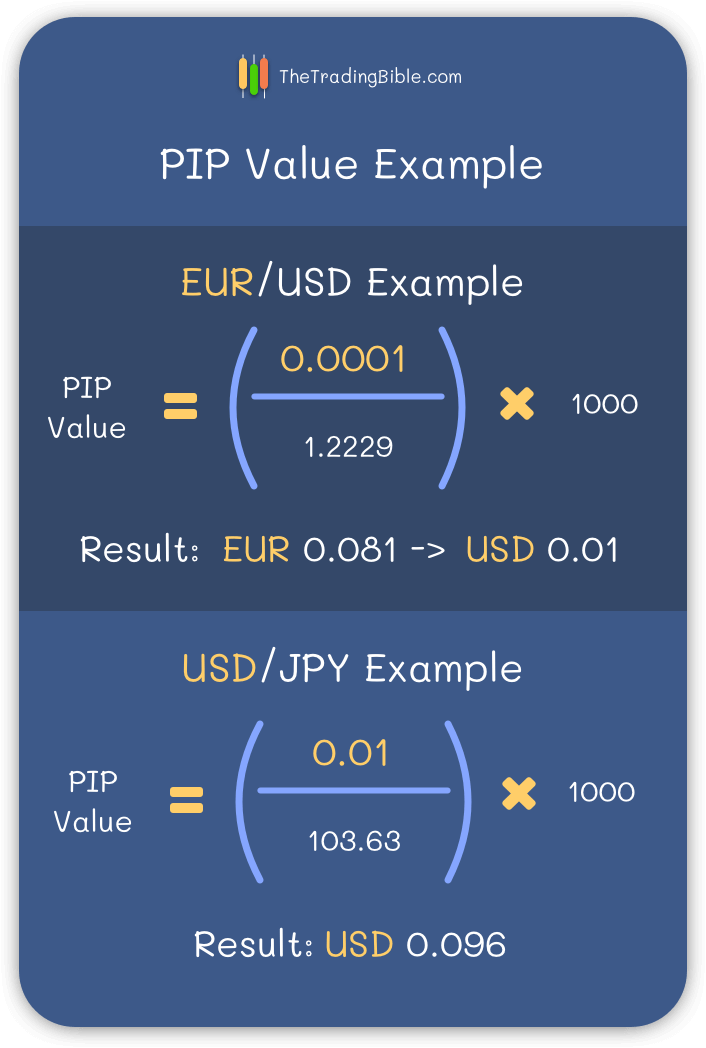Notice how in the first example, the result is expressed in Euros and then converted to Dollars, that happens because the formula always produces the result in terms of the base currency (the first one in your pair) and that’s why if you wish to see it in another currency you must convert it manually.

## Conclusion

Now that we learned that a Pip is simply a small measurement change in currency prices, we need to understand the logic behind all of this.